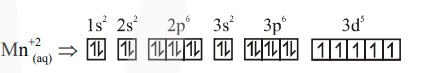Deepak Scored 45->99%ile with Bounce Back Crack Course. You can do it too!

# What is the spin-only magnetic moment value (BM) of a divalent metal ion with atomic number 25 , in it's aqueous solution?

Question:

What is the spin-only magnetic moment value (BM) of a divalent metal ion with atomic number 25 , in it's aqueous solution?

1. $5.92$

2. $5.0$

3. zero

4. $5.26$

Correct Option: 1

Solution:

Electronic configuration of divalent metal ion having atomic number 25 isTotal number of unpaired electrons $=5$

$\mu$ (Magnetic moment) $=\sqrt{\mathrm{n}(\mathrm{n}+2)}$ BM

where $n=$ number of unpaired $e^{-}$

$\therefore \mu=\sqrt{5(5+2)}=\sqrt{35} \mathrm{BM}=5.92 \mathrm{BM}$